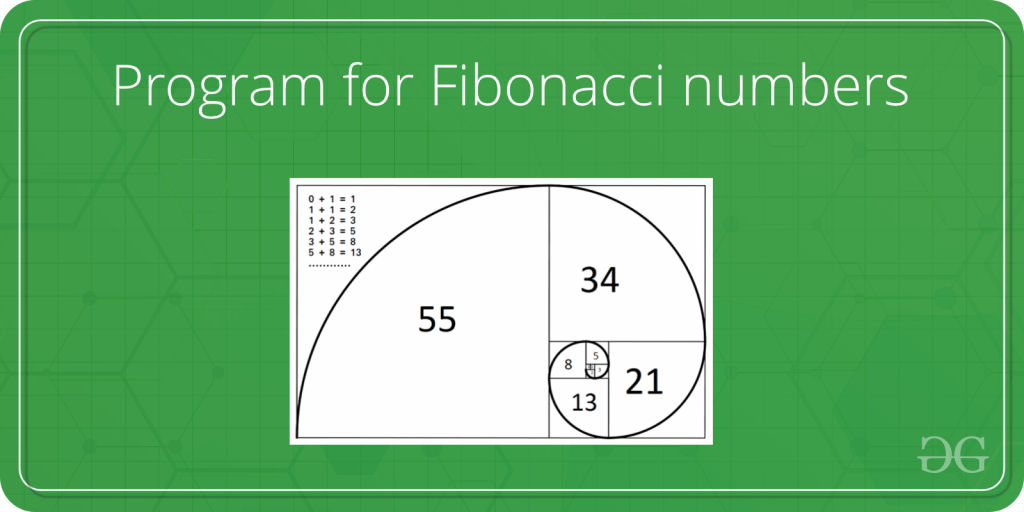Skip to content
Related Articles
Python | Find fibonacci series upto n using lambda
• Difficulty Level : Expert
• Last Updated : 17 Mar, 2019

The Fibonacci numbers are the numbers in the following integer sequence.

0, 1, 1, 2, 3, 5, 8, 13, 21, 34, 55, 89, 144, ……..

In mathematical terms, the sequence Fn of Fibonacci numbers is defined by the recurrence relation

Fn = Fn-1 + Fn-2  with seed values   F0 = 0 and F1 = 1.Find the series of fibonacci numbers using lambda function.

Code #1 : By using lambda and reduce method

 `from` `functools ``import` `reduce`` ` `fib ``=` `lambda` `n: ``reduce``(``lambda` `x, _: x``+``[x[``-``1``]``+``x[``-``2``]],``                                 ``range``(n``-``2``), [``0``, ``1``])`` ` `print``(fib(``5``))`
Output:
```[0, 1, 1, 2, 3]
```

Explanation :

The list taking first two parameters is 0 and 1, and add like x[-1] i.e 0 and x[-2] i.e 1 and append to variable x. There is a type conversion to list and due to `reduce()` method, the same function calls and due to range function this time parameter changes, then add this to previous result and again store it to list.

Code #2 : By using lambda and map function

 `def` `fibonacci(count):``    ``fib_list ``=` `[``0``, ``1``]`` ` `    ``any``(``map``(``lambda` `_: fib_list.append(``sum``(fib_list[``-``2``:])),``                                         ``range``(``2``, count)))`` ` `    ``return` `fib_list[:count]`` ` `print``(fibonacci(``10``))`
Output:
```[0, 1, 1, 2, 3, 5, 8, 13, 21, 34]
```

Explanation :
We are taking the list fib_list which already has 0 and 1. Then in the next iteration, this will be used as input and result of their sum will append to the list.

Attention geek! Strengthen your foundations with the Python Programming Foundation Course and learn the basics.

To begin with, your interview preparations Enhance your Data Structures concepts with the Python DS Course. And to begin with your Machine Learning Journey, join the Machine Learning – Basic Level Course

My Personal Notes arrow_drop_up